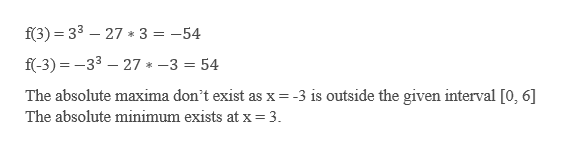# Find the absolute extrema of the function on the closed interval. Use a graphing utility to verify your results. (If an answer does not exist, enter DNE.)f(x) = x3 − 27x,    [0, 6]absolute maximum    (x, y) =        absolute minimum    (x, y) =

Question
85 views

Find the absolute extrema of the function on the closed interval. Use a graphing utility to verify your results. (If an answer does not exist, enter DNE.)

f(x) = x3 − 27x,    [0, 6]
absolute maximum     (x, y)  =

absolute minimum     (x, y)  =

check_circle

Step 1

Given a function

Step 2

To find: Absolute extrema of a function in closed interval [0, 6].

Step 3

Now, substitute the point...help_outlineImage Transcriptionclosef(3) 33 27 3 = -54 f(-3) 33 27* -3 54 The absolute maxima don't exist as x -3 is outside the given interval [0, 6] The absolute minimum exists at x 3 fullscreen

### Want to see the full answer?

See Solution

#### Want to see this answer and more?

Solutions are written by subject experts who are available 24/7. Questions are typically answered within 1 hour.*

See Solution
*Response times may vary by subject and question.
Tagged in

### Derivative